# XNOR Gate | A tutorial with the Truth Table and use cases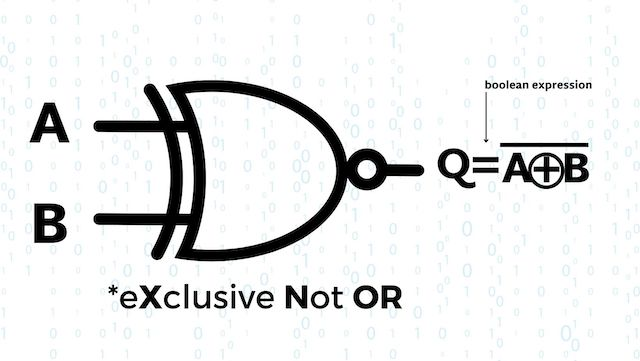An XNOR gate is a digital logic gate that produces a
high output (1) only if its two inputs are equal.

XNOR gates are commonly used in digital circuits to perform arithmetic and data processing operations.

## The XNOR (eXclusive Not OR) Gate

It’s a combination of the following

• XOR Gate: Produces a high (ON or 1) output if there is exclusively one high input
• and the NOT Gate: aka the inverter, which produces an output opposite to its input.

Thus the name, eXclusive Not OR Gate (XNOR)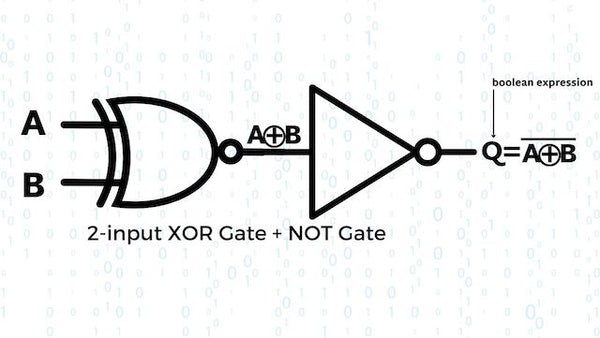That being said, XNOR has a truth table (more on Truth Tables later) similar to the NOR Gate. Meaning that its normal output level is high and goes low (OFF or 0) when ANY individual input is high (ON or 1). Simply put, if the inputs aren’t equal, the output will be low, so you’ll also hear it called the “Equivalency Gate”.

Fyi, the following terms are used interchangeably
1 | HIGH | ON
O | LOW | OFF

Let’s check out what the Truth Table can tell us about the XNOR Gate.

## Truth Table of the XNOR Gate

The truth table of an XNOR gate summarizes its behavior for all possible input combinations.

### 2 input XNOR Gate

As mentioned above, the 2 input XNOR Gate is naturally in a high state and will stay that way unless the inputs differ. If they’re both the same (high or low), a high output will be produced.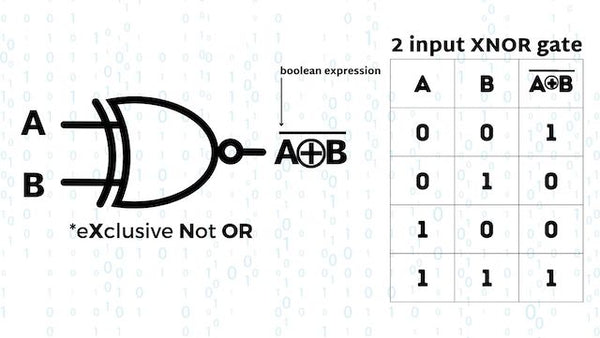Generally, XNOR will give a high output when there  are an even number of high inputs (1s). We say generally because the 2 input XNOR Gate does act a bit differently, let’s check it out.

### 3 input XNOR Gate

Then an Ex-NOR function with more than two inputs is called an “even function” or modulo-2-sum (Mod-2-SUM), not an Ex-NOR. This description can be expanded to apply to any number of individual inputs as shown below for a 3-input Exclusive-NOR gate.Here's what all the basic logic gates look like in a 2-input Truth Table.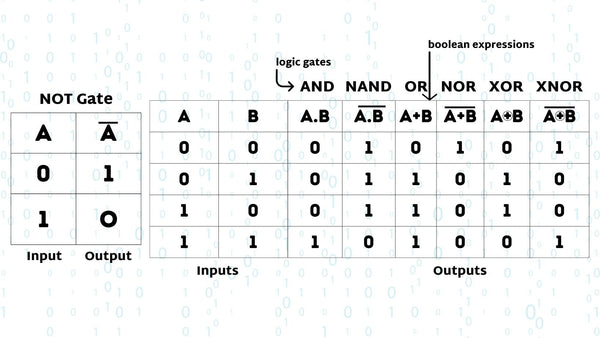## Applications of the XOR Gate

As mentioned, XNOR is the combination of XOR (eXclusive OR) and a NOT gate. Using the 2 input truth table we just showed you, here's how you can create an XNOR gate with a combination of other basic logic gates.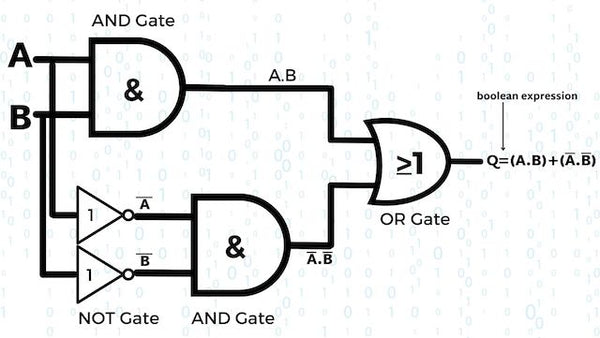The circuit above uses an AND, NOT, and OR gate to create XNOR, but it can also be created from NAND Gates.Here's what the pinout diagram of an IC 74266, a TTL quad-2 input XNOR Gate looks like. It contains four 2-input XNOR Gates in a 14-pin DIP.Now that we know what they are used for, how does XNOR compare with the other logic gates?

## Comparing XNOR with Other Logic Gates

As a quick overview, here are the main differences between XNOR and the other basic logic gates, like AND, OR, and NOT Gates.

• AND Gate: Produces a high output only when all of its inputs are at logic level high. In contrast, the XNOR gate produces a high output when both inputs are the same, either high (1) or low (0)
• OR Gate: Produces a high output when at least one of its inputs is at logic level "1." On the other hand, the XNOR gate produces a high output when both inputs are the same, either high (1) or low (0)
• NOT Gate: The NOT gate (also called an inverter) takes a single input and produces the complement of that input at the output. It reverses the logic level. In comparison, the XNOR gate requires two inputs and checks if they are the same, producing a high output only when they are equal.
• XOR Gate: Produces a high output when the inputs are different and "0" when they are the same. The XNOR gate, on the other hand, produces an output of "1" when the inputs are the same and "0" when they are different.
• NAND Gate: Produces an output of "1" unless all of its inputs are at logic level "1." In comparison, the XNOR gate produces an output of "1" only when the inputs are the same.
• NOR Gate: Produces a high output when all of its inputs are at logic level "0." Conversely, the XNOR gate produces an output of "1" when the inputs are the same, either "0" or "1."

## The XNOR Gate in Digital Logic | Final thoughts

Understanding the XNOR gate is an important step in understanding how digital devices process information, and it provides a solid foundation for further study in electronics and computer science.